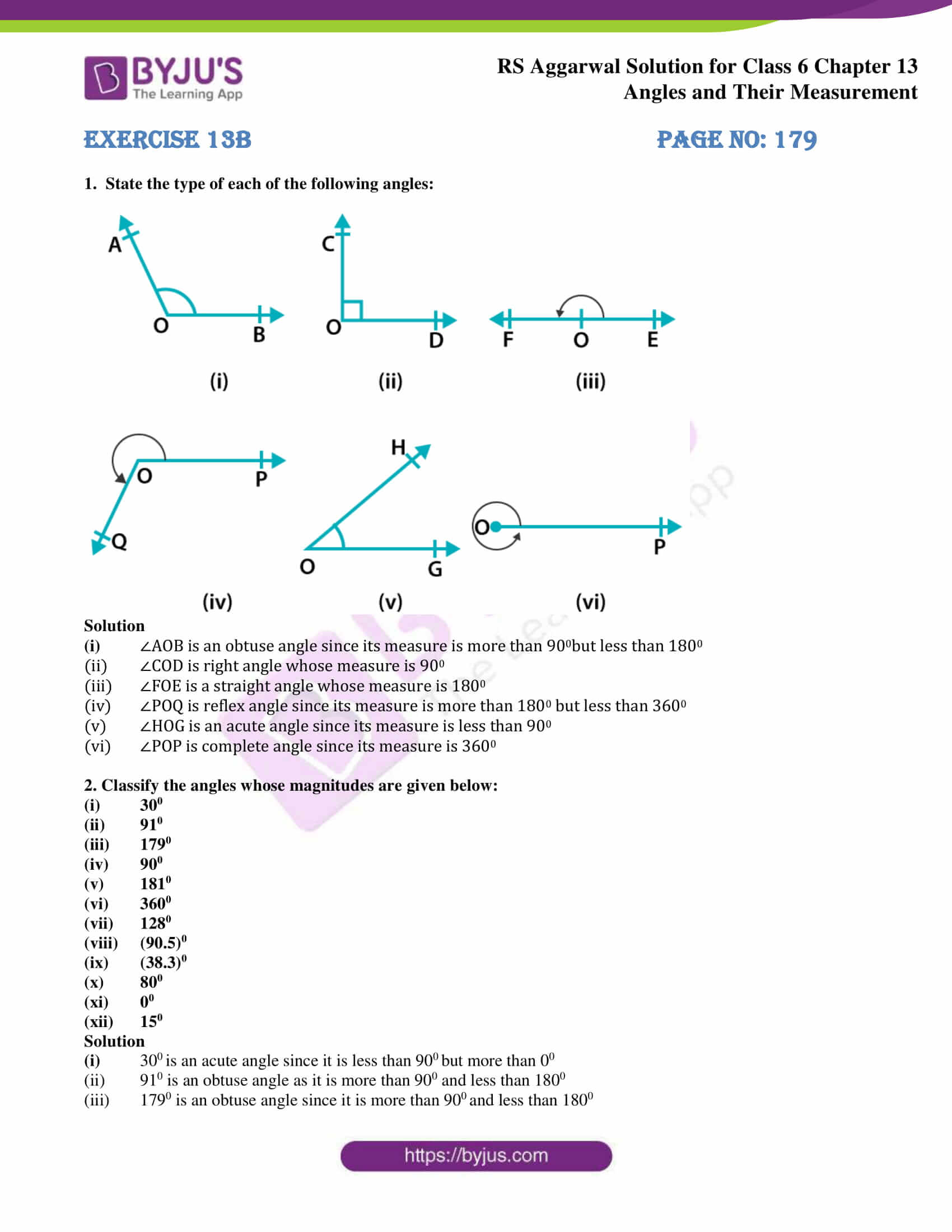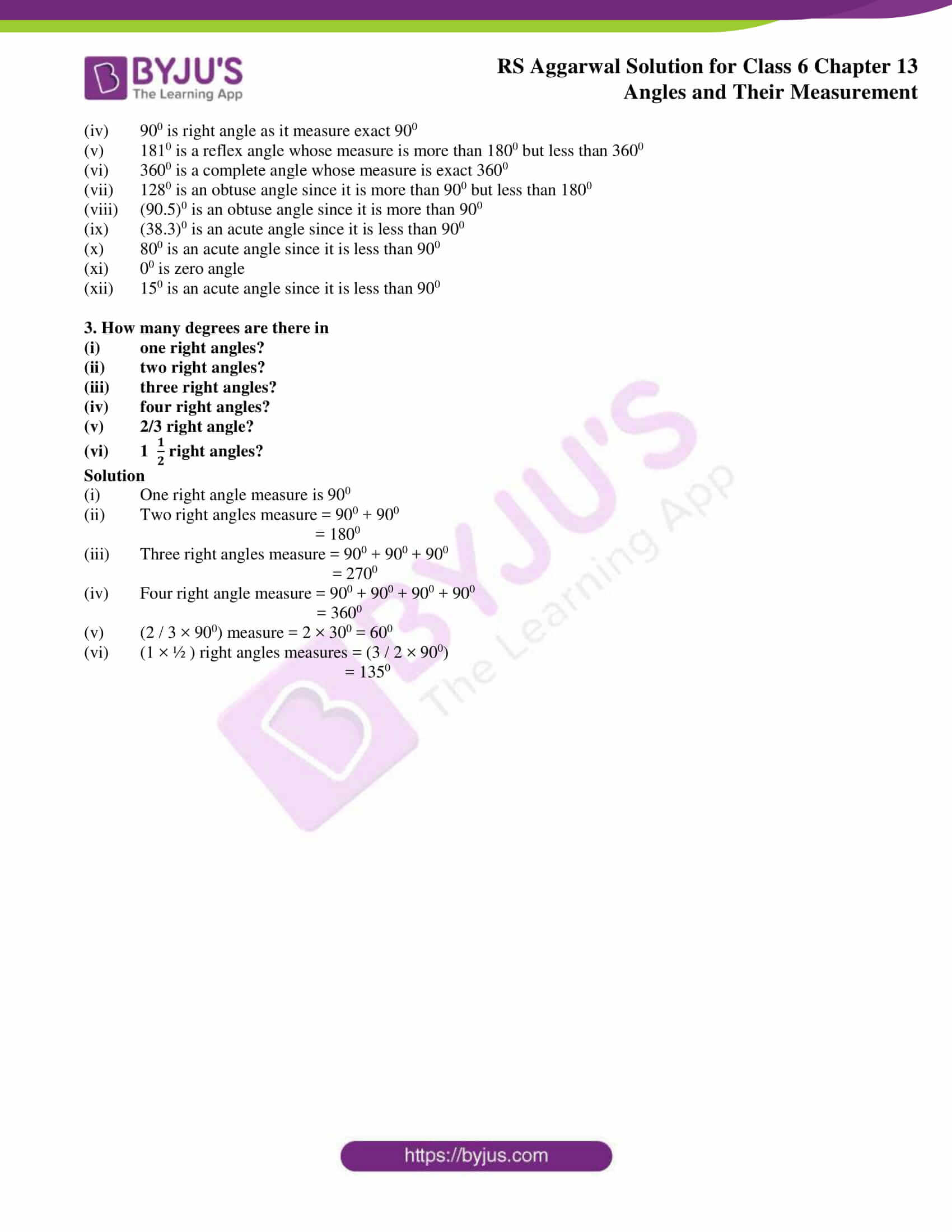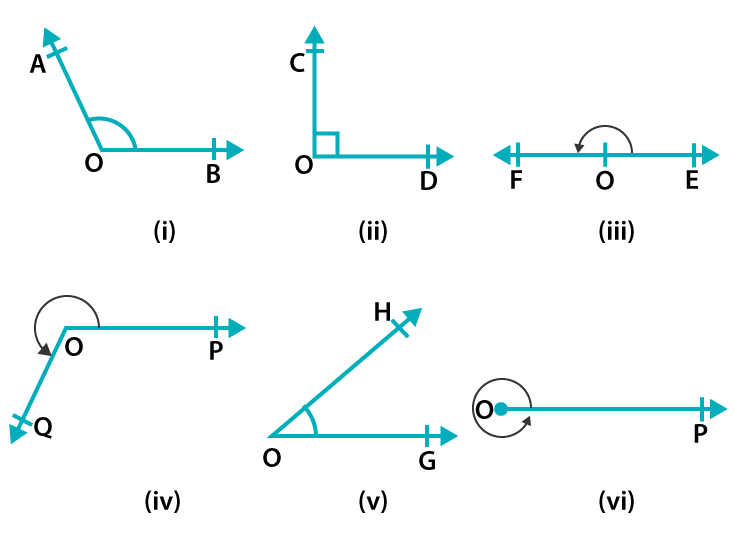# RS Aggarwal Solutions for Class 6 Chapter 13 Angles and their Measurement Exercise 13B

Mathematics is one of the subjects which requires more knowledge about the concepts to score well in the exam. RS Aggarwal Solutions created by faculty at BYJU’S help the students to get an overall idea about the topics. It is a vital resource for the students from exam point of view. The students can improve their self-study and problem-solving skills to have a good academic score. RS Aggarwal Solutions for Class 6 Chapter 13 Angles and their Measurement Exercise 13B PDF is available here.

## Download PDF of RS Aggarwal Solutions for Class 6 Chapter 13 Angles and their Measurement Exercise 13B### Access answers to Maths RS Aggarwal Solutions for Class 6 Chapter 13 Angles and their Measurement Exercise 13B

1. State the type of each of the following angles:Solution

(i) ∠AOB is an obtuse angle since its measure is more than 900but less than 1800

(ii) ∠COD is right angle whose measure is 900

(iii) ∠FOE is a straight angle whose measure is 1800

(iv) ∠POQ is reflex angle since its measure is more than 1800 but less than 3600

(v) ∠HOG is an acute angle since its measure is less than 900

(vi) ∠POP is complete angle since its measure is 3600

2. Classify the angles whose magnitudes are given below:

(i) 300

(ii) 910

(iii) 1790

(iv) 900

(v) 1810

(vi) 3600

(vii) 1280

(viii) (90.5)0

(ix) (38.3)0

(x) 800

(xi) 00

(xii) 150

Solution

(i) 300 is an acute angle since it is less than 900 but more than 00

(ii) 910 is an obtuse angle as it is more than 900 and less than 1800

(iii) 1790 is an obtuse angle since it is more than 900 and less than 1800

(iv) 900 is right angle as it measure exact 900

(v) 1810 is a reflex angle whose measure is more than 1800 but less than 3600

(vi) 3600 is a complete angle whose measure is exact 3600

(vii) 1280 is an obtuse angle since it is more than 900 but less than 1800

(viii) (90.5)0 is an obtuse angle since it is more than 900

(ix) (38.3)0 is an acute angle since it is less than 900

(x) 800 is an acute angle since it is less than 900

(xi) 00 is zero angle

(xii) 150 is an acute angle since it is less than 900

3. How many degrees are there in

(i) one right angles?

(ii) two right angles?

(iii) three right angles?

(iv) four right angles?

(v) 2/3 right angle?

(vi) 1 right angles?

Solution

(i) One right angle measure is 900

(ii) Two right angles measure = 900 + 900

= 1800

(iii) Three right angles measure = 900 + 900 + 900

= 2700

(iv) Four right angle measure = 900 + 900 + 900 + 900

= 3600

(v) (2 / 3 × 900) measure = 2 × 300 = 600

(vi) (1 × ½ ) right angles measures = (3 / 2 × 900)

= 1350

### Access other exercises of RS Aggarwal Solutions for Class 6 Chapter 13 Angles and their Measurement

Exercise 13D Solutions